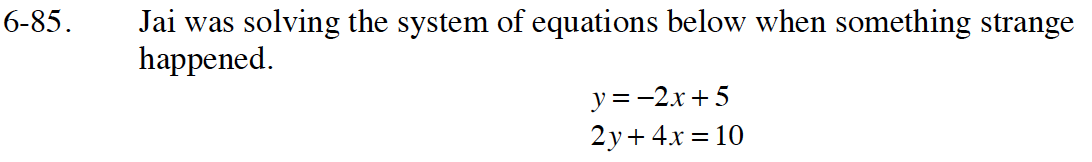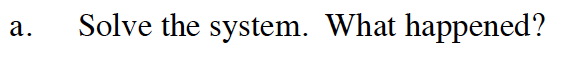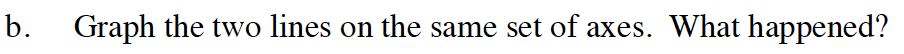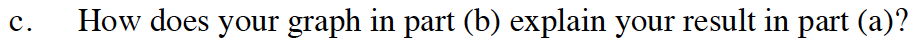Home > GB8I > Chapter cc46 > Lesson cc46.3.1 > Problem6-85

6-85.
1. Jai was solving the system of equations below when something strange happened. 6-85 HW eTool (Desmos). Homework Help ✎

 y = −2x + 5 2y + 4x = 10
1. Solve the system. What happened?

2. Graph the two lines on the same set of axes. What happened?

3. How does your graph in part (b) explain your result in part (a)?Use the substitution method and solve for x.

x = ARN (All Real Numbers)Put the equations in the y = mx + b form.What does the graph show about the points of intersection these lines have?

Use the eTool below to help solve the problem.
Click the link at right for the full version of the eTool: Int1 6-85 HW eTool